# Container Algebra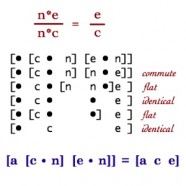The arithmetic of Container Numbers can be generalized to an algebra based solely on the ideas of putting together (addition/subtraction as merging containers) and putting into (multiplication/division as substitution), together with a generic modifier that creates negative numbers. This iconic algebra is significantly simpler than our conventional algebra based on sets and group theory, while maintaining all the advantages of physically grounded iconic math. The Image shows a proof of the reducibility of fractions using only the iconic definition of multiplication and division as substitution. This page presents aspects of the formal theory of iconic container numbers. How iconic math works when it is applied to numbers is specified by equations that are usually called rules. The axioms of iconic algebra are first compared to the conventional axioms of symbolic algebra. Then the rules of equality, merging, and substitution are described.

## Iconic and Symbolic Axioms

Unit-ensemble arithmetic is the archetypical iconic system that illustrates axiomatic differences between spatial and symbolic computation, and thus between embodied and rote mathematics education. Ensembles are base-1, so there is no concern with managing place-value or depth-value notation. The zero place-holder that supports positional notation does not exist in unit-ensemble arithmetic. The capabilities to put together and to substitute into formally define how both addition and multiplication work. These two computational mechanisms, substitution and juxtaposition, are assumed in almost all algebras, whether symbolic or iconic. One difference is that symbolic systems separate computation from concept, thus requiring further axioms to define the algebra itself. Iconic systems define the computational mechanisms as the algebra. Thus, the process of iconic computation shows mathematical concepts in action, while the conceptual structure of an iconic mathematics defines the activity of using those concepts.

Another difference is that ensembles permit arithmetic to proceed in a parallel (space-like) rather than a sequential (time-like) manner. This renders the conventional rearrangement axioms of Commutativity and Associativity irrelevant. Ensembles are also free from the idea that only two numbers can be operated upon at the same time (the concept of binary arity). Many iconic numbers can be added together at the same time.

The four structural axioms of unit-ensemble arithmetic are Merge, Cancel, Commute, and Distribute. These four axioms define addition as deletion of spatial partitions, subtraction as annihilation of polar units, and both multiplication and division as substitution. The iconic axioms of unit-ensembles have an intuitive grounding in simple physical actions.  The notation may be unfamiliar, but what the notation says to do is familiar to first grade students. Each is examined later in the page.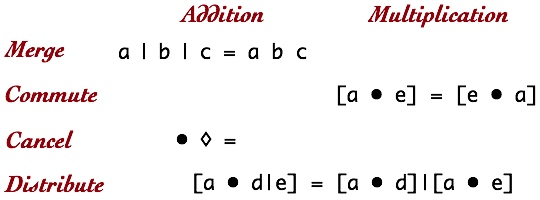Compare the four iconic axioms to the nine symbolic axioms that are currently taught.  The symbolic axioms represent a particular way of thinking.  They anchor arithmetic to the abstract mathematical theory of groups.  Group theory is immensely valuable for higher math, but it is not commonly known, it is not relevant to almost all non-mathematicians, and it does not help those trying to learn practical arithmetic or algebra.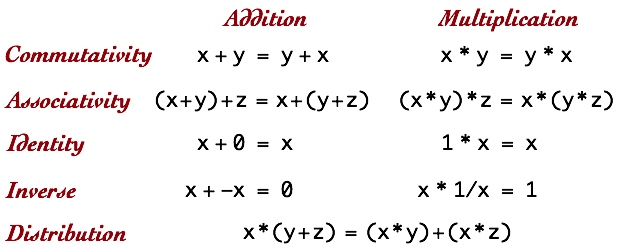A deep problem with these axioms is that they do not define addition or multiplication. They do not show us what to do to add or to multiply, and they do not tells us what addition or multiplication mean. These group theoretic axioms tell us something much more abstract, they identify invariant structures within transformation systems. Of course we would expect our common number system to be part of a larger grand mathematical structure, but this grand structure is relatively new, undergoing constant evolution, and most importantly, very poorly understood by pre-college mathematics teachers. The situation is quite analogous to having to understand how to build a turbine engine in order to board an airplane.

It is not uncommon for tools and ideas to have multiple meanings and multiple uses. Math education has abandoned the most common use of numbers in favor of an orthodoxy of belief in the theory of groups. This is not particularly surprising, since this two hundred year old theory completely changed the face of mathematics. Math classes pay homage by having young students go through exercises that are without historical context, without conceptual grounding, and without practical utility. Worse though, this abstract perspective robs students of their native intuitive understanding of the other meaning of numbers as convenient tools that have evolved over thousands of years. Unfortunately, suppression of the common sense alternative leads teacher training textbooks to engage in awkward explanations that undermine even the teachers’ understanding of how common arithmetic works by explaining the meaning of addition in terms of symbol manipulation rather than in terms of the spatial intuitions of the Additive Principle.

## Equality

What can we do with arithmetic expressions that does not change their value or meaning?  What can we do when we know that two expressions mean the same thing?  In iconic algebra equality is defined by the substitutions that do not change meaning.  Generally, substitution of, for, and into equals achieves computation.

Our notation for substitution is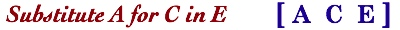The statement Substitute A for C in E  describes a compound action that begins by identifying two syntactic forms, A and E.  The E form will be changed by at least one insertion of the A form into the body of the E form.  Whenever a C form is identified with E, it is converted into an A form.  A significant question is whether or not all C forms must be replaced.  We will assume yes.

The validity of a substitution is judged in relation to the meaning of E, which must not change even while the literal form of E is changing.  The appropriateness of a substitution, however, is judged in relation to the syntactic form since substitutions are usually goal-driven, with  maintenance of meaning acting as a constraint rather than as a strategy.  The following constraints are the tools of the substitution process.

When reading the following shorthand notation, remember that each substitution calls for an action, something that a student can do or is doing.  We begin with cases for which substitution has no effect.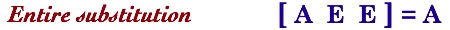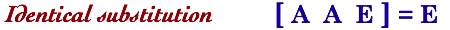The first statement asserts that substituting A for an entire form E leaves A.  The second statement asserts that substituting A for itself does not change anything.  Next, the rule of Replacement: substituting A for some other form C does not change the meaning of E only when A=C.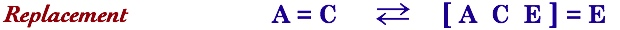Finally, there are exactly three cases when a substitution does not change the value of E.

When two forms are equal, it doesn’t matter which one is substituted into E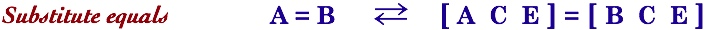When two forms (within E) are equal, it doesn’t matter which one is substituted for.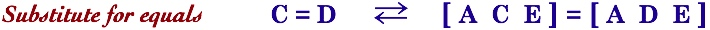The same substitution into two equal forms keeps them equal.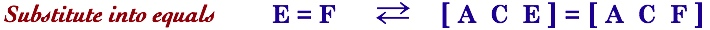We’ll return to substitution in a moment in the context of multiplication and division.

## Formal Iconic Math

Iconic addition calls upon the Additive Principle, however putting together does not formally characterize how addition works, so a comparison to the formal structures of group theory is somewhat unfair. Formal math is synonymous with symbolic math, math that can be performed without error or ambiguity by a computer following clear algorithmic steps. The opposite of how people think. It is the desire for incontrovertible proof that has led mathematics and math education to abandon humane, intuitive math. To be considered as mathematical, it’s a necessary prerequisite that iconic math, as well as being visual and interactive, must also be formal. It is.  Substitution is well accepted as a fundamental part of the formal toolset so, for example, iconic multiplication by substitution is naturally formal.

How can the additive principle be expressed in a language suitable for computation and for acceptance as legitimate mathematics? One approach is to look toward mereology, the formal study of whole/part relationships. Technically a unit-ensemble is a mereological whole with parts that do not support overlap.

## No Zero

Mereology has the necessary concept structure for iconic addition. Unlike formal addition which relies at its foundation upon the concept zero (stating that zero is a number and that no addition can result in zero), both mereology and iconic arithmetic have no zero concept. An ensemble without parts does not exist. Unlike the conventional zero which must exist at some location, absence exists everywhere throughout an iconic number. In particular, an iconic container can contain nothing at all. Spencer Brown Numbers for example, use hollow enclosures as units, so that other forms can be placed inside a unit, effectively turning the unit into an operator.  The units we have been using for unit-ensembles do not have an inside. Absence also occurs as a result of the Cancel Rule.  When a positive and a negative unit are put together, both cease to exist. Finally, it is possible to substitute nothing for some existing structure. We usually call that deletion or erasure.  Technically it is void-substitution.

## Merging

In formal mereology, parts are composed together into wholes. Each whole is exactly the sum of its parts, and nothing more. Mereology defines fusion as the method by which ensembles are constructed. For example, the fusion of ••• with •• is •••••. When ensembles are defined by enclosing containers, the mereological concept of fusion can be replaced by a simpler concept of merging containers. The advantage of expressing addition as merging containers is that merging is an action preformed on containers themselves, independent of their contents. We introduce two notations for merging, one is iconic, the other typographical, with the usual caution is that typographical notation introduces incidental, spurious structure into the representation of iconic concepts.

Merging is the deletion of separating boundaries. Here is an illustrative example, recorded both in space and in a line.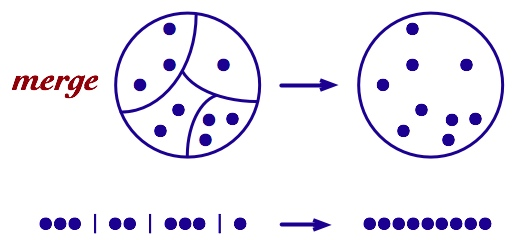The general merging rule can be stated algebraically.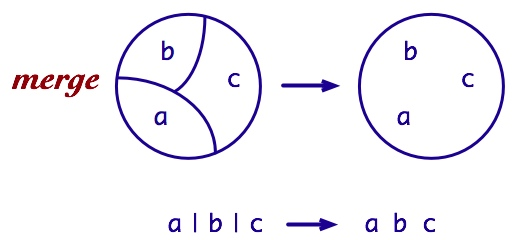Merging has three important properties in common with the informal idea of putting together. Merging is independent of the size of the ensembles being merged. The act of putting together does not depend on exactly what is being put together. Merging is not limited to a specific number of ensembles being merged. The contents of any number of containers can be put together at the same time. Finally, merging is an action, not a symbolic substitution.

The boundaries that are eliminated during merging do not necessarily need an interpretation or meaning. It suffices that they simply differentiate or bound. The separating bars in the textual notation are meant to identify an initial differentiation or distinction, an assignment of units to different containers. Merging removes that initial distinction. If we use a white lenticular bracket to represent imagined or real boundaries/distinctions, then merging can be expressed as collection into one container by deleting container boundaries.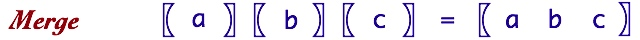## Substitution

Once substitution is used to define multiplication, it also defines division, fractions and exponents.  Multiplication is defined as substitution of an ensemble for each unit within another ensemble.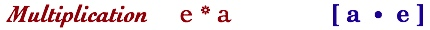Although it is ambiguous which variable is multiplying which, we will adopt the convention that e is multiplied by a, so that the intermediate result would be a copies of e. When interpreted as numerical multiplication, substitution is commutative. This leads to quite different ways to interpret both substitution and multiplication.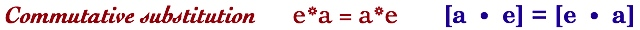The form a can be substituted into e, or e can be substituted into a, without changing the result.  Almost always, the representation of the results of each substitution will look different, but the value or meaning of the two will remain the same.  Conventionally, a*e=e*a.  The example 3*2=2*3 is commonly illustrated as two rectangles with replicates of the number being multiplied stacked either horizontally or vertically.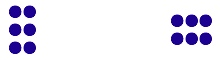This visual not only expresses multiplication as explicit replication, it also relies upon the orientation of the rectangles on the page to convey the difference of which number is multiplied by which.  This common explanation of multiplication confounds viewing perspective with commutativity, which is essentially permission to ignore ordering and not a relationship between the position of the observer and the result of the multiplication.

For iconic multiplication as substitution, putting 2 into 3 must yield the same result as putting 3 into 2. The resulting forms are each equal to 6 while the representation of 6 achieved by each substitution differs structurally rather than spatially.  (Animations of these substitutions are in Container Numbers.)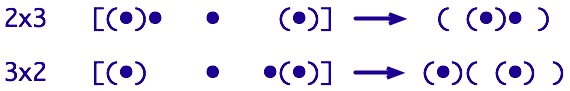Since substitution provides the meaning of multiplication, the structural difference between which form is substituted into which identifies a difference in efficiency. In the conventional rectangular layout this correspondes to the number of replication steps. For example, replicating two three times, or replicating three two times. Conventionally, mathematics pays little attention to the level of effort required to achieve an operation; effort is the domain of Computer Science. One consequence is that mathematics pays little attention to the difference between sequential and parallel algorithms. Computational effort is also the domain of education, where the difficulty of both learning and performing an algorithm does matter to students. A fundamental perspective of iconic math is that conceptually some operations are necessarily parallel and some operations are simpler than others, so ignoring how a computation unfolds is misleading for both effort and for understanding.

### Associative Substitution

The composition of two binary multiplications is associative. Conventionally, A*(B*E) = (A*B)*E. In substitution, associativity is an assertion that the order of doing substitution does not matter.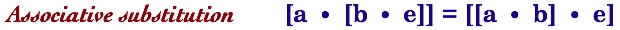As illustrated on the Parens Notation page, the order of substitution matters if you are working with paper and pencil as most students are. Another refinement that is well-studied in Computer Science is whether to fully reduce the first substitution prior to performing the second (applicative order) or to do all the substitutions, then reduce (normal order). The point is that conventional multiplication provides no information about effort, while multiplication as substitution provides explicit information. Pragmatically, in computing 5*6*7, it is less difficult to multiply 30*7 than to multiply 5*42.

Commutativity and associativity lead to flat substitution for which, like multiplication, the ordering of substitutions does not matter (i.e. ordering is a matter of convenience). Flat substitution means we can remove the inner brackets, since it does not matter which substitutions occur when.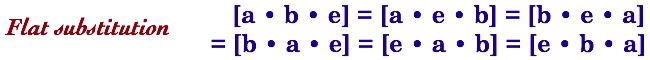Since forms are substituted for units there is a clear criterion to minimize effort, to substitute first into forms with the least number of units. Flat substitution also provides a clear image of exponential powers. To square a number, substitute the form of that number for every unit in itself.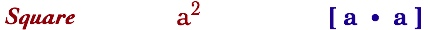Higher powers follow the same pattern.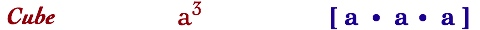At this point, it is apparent that a more powerful notation is needed for higher exponents, as well as for roots and logarithms. This brief introduction will not include those extensions, however James Numbers provide an elegant and substantively different iconic notation for exponents and logarithms.

The structure of safe substitution — substitution that does not change the value of the form substituted into — is described in the Equality section above. Flat substitution collapses several of these distinctions. The two degenerate cases, Entire and Identical Substitution, become one. Substitution of Equals and Substitution into Equals are also the same thing. The flexibility of flat substitution becomes most apparent when we consider division, but before doing so, here is an interesting visualization of the relationship between substitution and powers.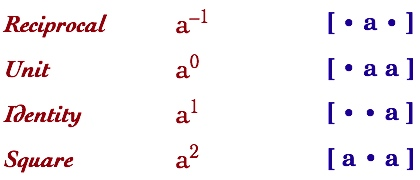### Division by Substitution

Division is defined as substitution of a unit for every divisor within a form. Here we will consider only simple cases of exact division. Extension to quotients with remainders, reciprocals and fractions is relatively easy, however the notation and the type of thinking can vary significantly from what we have all learned as the way to think about division and fractions.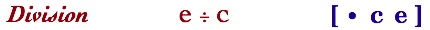Flat substitution extends to the included division. We first identify the structure of a reciprocal. The reciprocal of a number c is formed by substituting a unit for every c in a unit.  That is, a unit fraction is the subdivision of the unit into the number of parts equal to the “denominator”.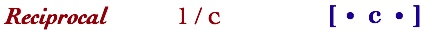Before considering what fractional substitution means, we can observe that flat multiplication already incorporates the concept of a reciprocal within the middle term of these equivalent substitution sequences.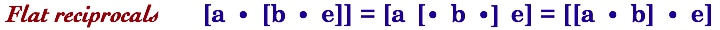Flat substitution asserts that any three contiguous forms constitute a valid substitution pattern. The middle substitution above shows a third interpretation of what it means to multiply three numbers together. That is, we can substitute a for the reciprocal of b in e. Conventionally we might write this as (a*e)/(1/b). This reading of multiplication is unusual in standard notation but overt in the notation of flat substitution. Further, due to commutativity of substitution, any forms in odd positions in a flat substitution can be exchanged with one another, while any forms in even positions can also be exchanged with one another.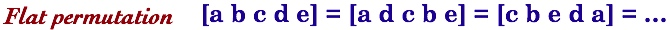Although it is obvious that sequential multiplication and division can be performed in any order, it is less obvious for substitution sequences.

A simple division by substitution, 6/2, is animated in Container Numbers. Here it is shown as a substitution process for unit-ensembles. The ensembles that will be replaced by units are identified by partitioning 6, a process analogous to successive subtraction. The substituted unit is highlighted as an asterisk, *.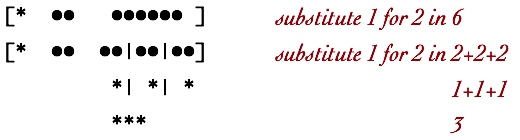### Substitution with Types of Units

In Unit-ensembles, negative numbers are integrated with positive numbers by identifying a negative unit, . The Cancel Opposites Rule permits subtraction while maintaining the Additive Principle. Negative units however play a different role in the process of multiplication, so that cancelation is insufficient. We need to be able to express (–1)*(–1)=1 via substitution. Substitution of negative units works well for most but not all substitution patterns. The particular issue is the form [• ◊ •], which reads “substitute for in “. The difficulty is that there are no s in , so we would expect no substitutions to occur. Another issue that was finessed for unit-ensembles was the notation for large negative numbers. Both issues can be addressed by introducing a modifier that works just like the negative sign, –. In order to not confuse common notation, we’ll introduce delta, , in place of the object to represent a negation operator.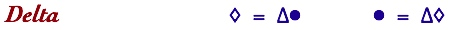The operator can entirely replace the unit. As a modifier rather than an object, delta acts upon units, without consideration of a negative domain. The new Cancel Rule is more general since delta can apply to any ensemble.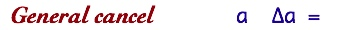Delta now provides the structure for multiplying negative numbers while maintaining an Additive Principle that achieves subtraction. Multiplication of “negative numbers” is expressed by a self-canceling property.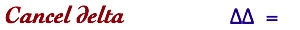The prominent use of cancelation into nothing (technically, void-substitution) is typical of iconic systems. The general Cancel rule illustrates the void-substitution associated with the delta operator. Collect Delta is very much like a Dirac Delta function, except that the two states of the Dirac function are 1 and 0, while the two states of the iconic delta are exists and does-not-exist. Let’s represent the presence or absence of a by a subscripted delta, 1.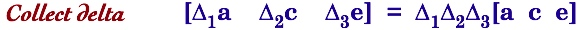The unit Cancel rule can now be written without the negative object, , thus associating the negative concept for addition with the negative concept for multiplication.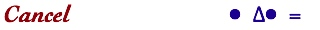Collect Delta permits us to show that (–1)*(–1)=1 via substitution.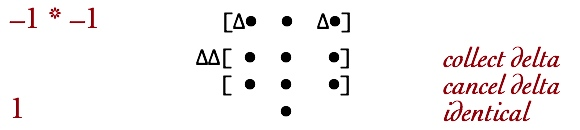### Fractions

The substitution form of the reciprocal 1/a is [• a •]. Read as substitution we encounter an immediate problem in interpretation. How can we substitute into a unit? This is essentially the same problem encountered by elementary school students when first encountering fractions. There are many clever ways to illustrate fractions to students (e.g. pieces of a pie), but most of these techniques fail to make it clear that the whole being subdivided was originally a unit. Our society in general protects us from unusual fractions, that is, from unusual subdivisions of a whole. An hour, for example is pre-subdivided into 60 minutes, so that 1/3 of an hour is not a fraction of an hour, but is 20 minutes instead. This idea guides us to an interpretation of substitution into a unit.

Fractions posit that units can be subdivided into pieces. The original unit is no longer unitary, but is rather a collection that is being partitioned. For the simplest unit fraction, 1/2, a unit is recast as two units, one of which is then used. What would this look like when phrased as a substitution?

When units are grouped together, say into 10s as in the decimal system, we construct a new form to represent that group. The idea is the same for fractions. The fraction 1/2 posits that the unit we are currently identifying is actually a group of two. We “borrow” against the original unit’s wholeness with the intention of reconstructing the original base of wholeness later. Fractions are just another way to express the base system of a number. Students have difficulty adding unlike fractions because that operation is analogous to adding two whole numbers expressed in different base systems. For addition to work, the base system of the two numbers must match.

#### Multiplication of Fractions

Multiplication of fractions is easy to understand because fractions themselves are a form of multiplication. We might say that there are two separate worlds of arithmetic, addition and multiplication (in group theoretic terms, the two operations of a field). In iconic terms, the world of merging and the world of substitution. Fractions are creatures of the substitution world. As a consequence they are easy to multiply and difficult to add. Here is the general rule for multiplying fractions, expressed as substitutions.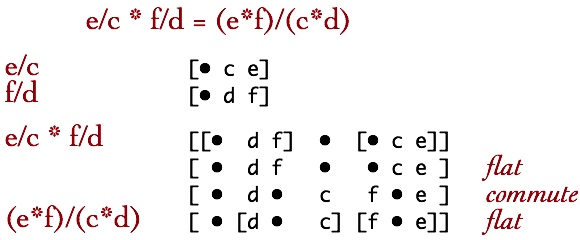Although the mechanism for multiplying fractions is straight-forward, a conceptual understanding of what it means to multiply fractions is more difficult, particularly for students who are provided only with the mechanism (i.e. multiple numerators by numerators and denominators by denominators). The substitution sequence shows why this works, we can commute substitutions. The general demonstration of multiplication of fractions above provides a behavioral roadmap. The originally prescribed substitutions call for finding d in f, and for finding c in e. Via commuting substitution this can also be achieved by substituting d into c and substituting f into e, and then finding the first result within the second. So multiplication of fractions can be explained as a choice between substituting twice then finding once or finding twice then substituting once. Here’s a concrete example. Imagine three friends who want to split one-half of a pizza. Cutting the pizza is division and is identified with the denominator of each fraction, while picking up one’s share is multiplication and is identified with the numerators. The right-hand-side of the multiply fractions rule conceptualizes the pizza as a whole (i.e. multiply the numerators) and then cuts it into six pieces (i.e. multiply denominators), with each friend picking up one of those pieces. The left-hand-side first cuts the pizza in half, and then cuts that half into three pieces. The half-pizza here is conceptualized as a whole. Presumably it is easier to cut than to calculate. The iconic substitute/find model identifies the act of cutting with finding parts, and substitution with what each person gets. Thus the left-hand-side requires us to find one-half of the pizza and then to find one-third of what remains. The right-hand-side requires us to find the whole pizza and then cut it into six pieces. Just making the distinction between the meaning of each side of the multiply fractions rule is tricky for young students.

#### Reduction of Fractions

Fractions can often be reduced, for example 4/8 = 1/2. In general, reduction can occur when the numerator and the denominator share a common multiple, (n*e)/(n*c) = e/c. We can prove that this works while identifying the substitution structure that facilitates reduction of fractions. Reduction involves two applications of Identical Substitution.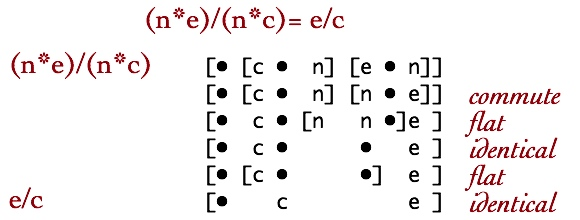Reduction of fractions could be expressed as a general pattern rule.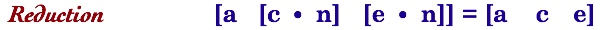#### Division of Fractions

Comprehension of what dividing fractions might mean is difficult.  Here is a very simple example, what could 1/2 ÷ 1/2 possibly mean?  We can try to understand the dynamics of dividing fractions by observing the iconic structure of the substitution sequence. That dynamics is exposed via flat substitution, the ability to identify a substitution act with any three contiguous forms.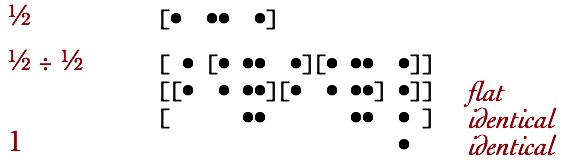We can first observe that there is no mysterious “Turn it upside down and multiply”. Using flat substitution, we can see that dividing a fraction by itself is an example of Identical Substitution, [A A E] = E, as it should be for self-division.

Here is an example of a slightly more complicated division using specific fractions to illustrate the construction of specific substitutions that achieve the desired division. This example also illustrates that conventional numerals can be used within the substitution calculus.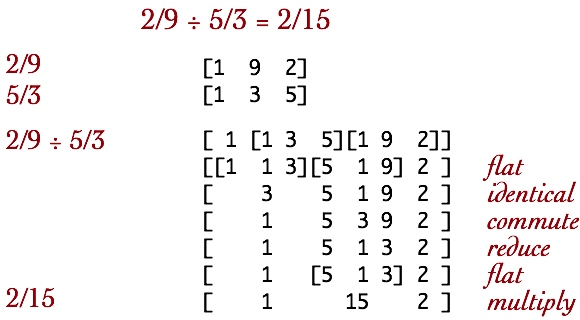This computation begins by applying Identical Substitution.  Then odd locations are rearranged to prepare for a reduction using the general reduction transformation shown above. Finally a multiplication is performed.  Like common fractions, the result is a compound form, a substitution to be made rather than a division to be made.

Here is the general process of dividing fractions.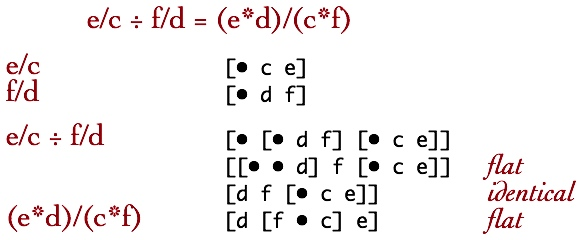Here is the general case of adding two fractions via substitution. The guiding principle is distribution of merging, since merging is the addition concept for substitution.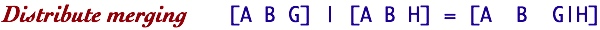We wish to distribute merging over substitution to arrive at a single substitution structure. To distribute, any two of the three substitution forms must match in both substitutions being distributed. The substituted form, , already matches. So the first step after transcription is to convert both middle forms, c and d, to a common substitution structure, [c • d]. Distribution of merging then completes the “addition”.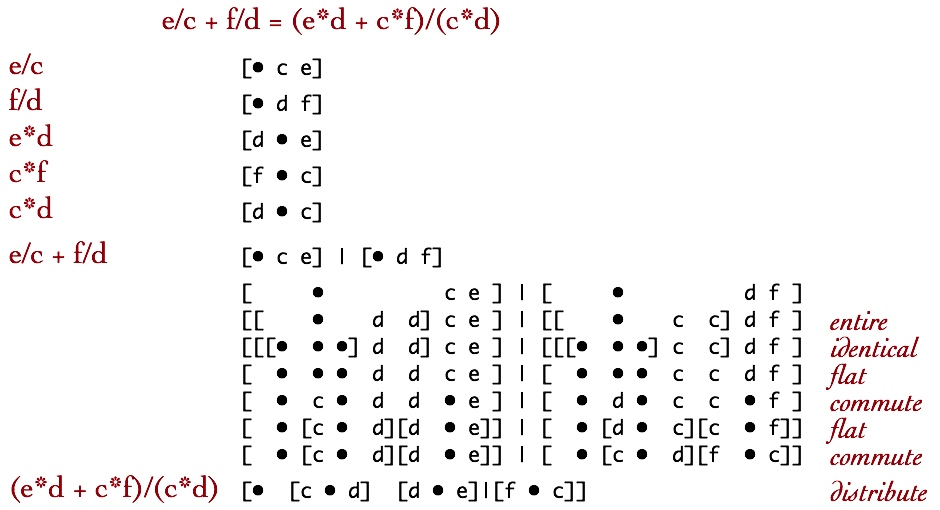#### Subtraction of Signed Fractions

The final example illustrates that delta provides the necessary conceptual basis for an integrated approach to both adding and multiplying negative numbers.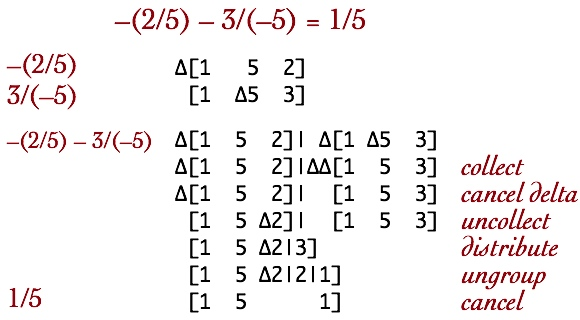The three uses of the negative sign in the conventional expression each convert to a delta operator.The Uncollect action is necessary since distribution is limited to substitution structures that are identical but for one element.

Yes, this is exotic! No, it is not intended to replace our common notation for fractions. The exercise is to demonstrate that it is possible to conceptualize all of elementary arithmetic as substitution processes. One gain is that we can consider elementary math not as a conglomerate of many different operations (+, , *, ÷, ^), but as varieties of only two operations, Merge and Substitute. The primary motivation is that these two operations maintain the Additive Principle. It is possible to simplify arithmetic on formal iconic grounds, without abandoning the intuitions of the Additive and the Multiplicative Principles.

Societally, each of us has spent at least five years learning how to do common arithmetic. In the US we know that the vast majority of us come out of the process both traumatized and unable to do arithmetic. The thesis of iconic algebra is that at least part of the problem is our mathematics itself.